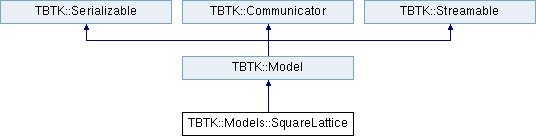TBTK Need a break? Support the development by playing Polarity PuzzlesTBTK::Models::SquareLattice Class Reference

Square lattice model. More...

#include <SquareLattice.h>

Inheritance diagram for TBTK::Models::SquareLattice:## Public Member Functions

SquareLattice (const Index &size, const std::vector< std::complex< double >> &parameters)Public Member Functions inherited from TBTK::Model
Model ()

Model (const std::vector< unsigned int > &capacity)

Model (const Model &model)

Model (Model &&model)

Model (const std::string &serialization, Mode mode)

virtual ~Model ()

Modeloperator= (const Model &rhs)

Modeloperator= (Model &&rhs)

void addModel (const Model &model, const Index &subsytemIndex)

int getBasisIndex (const Index &index) const

int getBasisSize () const

void generateHoppingAmplitudeSet (const HoppingAmplitude::AmplitudeCallback &hoppingAmplitudeCallback)

void generateOverlapAmplitudeSet (const OverlapAmplitude::AmplitudeCallback &overlapAmplitudeCallback)

void construct ()

bool getIsConstructed ()

void setTemperature (double temperature)

double getTemperature () const

void setChemicalPotential (double chemicalPotential)

double getChemicalPotential () const

void setStatistics (Statistics statistics)

Statistics getStatistics () const

const BasisStateSet & getBasisStateSet () const

const HoppingAmplitudeSetgetHoppingAmplitudeSet () const

const SourceAmplitudeSetgetSourceAmplitudeSet () const

const OverlapAmplitudeSetgetOverlapAmplitudeSet () const

GeometrygetGeometry ()

const GeometrygetGeometry () const

void createManyParticleContext ()

ManyParticleContext * getManyParticleContext ()

void setFilter (const AbstractHoppingAmplitudeFilter &hoppingAmplitudeFilter)

void setFilter (const AbstractIndexFilter &indexFilter)

Modeloperator<< (const AbstractState &basisState)

Modeloperator<< (const HoppingAmplitude &hoppingAmplitude)

Modeloperator<< (const std::tuple< HoppingAmplitude, HoppingAmplitude > &hoppingAmplitudes)

Modeloperator<< (const HoppingAmplitudeList &hoppingAmplitudeList)

Modeloperator<< (const SourceAmplitude &sourceAmplitude)

Modeloperator<< (const OverlapAmplitude &overlapAmplitudeSet)

virtual std::string toString () const

std::string serialize (Mode mode) constPublic Member Functions inherited from TBTK::Serializable
template<>
std::string serialize (const bool &data, Mode mode)

template<>
std::string serialize (const double &data, Mode mode)

template<>
std::string serialize (const std::complex< double > &data, Mode mode)

template<>
std::string serialize (const int &data, Mode mode)

template<>
std::string serialize (const unsigned int &data, Mode mode)

template<>
std::string serialize (const SpinMatrix &data, Mode mode)

template<>
std::string serialize (const Statistics &data, Mode mode)

template<>
std::string serialize (const std::vector< std::complex< double >> &data, Mode mode)

template<>
int deserialize (const std::string &serialization, Mode mode)

template<>
unsigned int deserialize (const std::string &serialization, Mode mode)

template<>
double deserialize (const std::string &serialization, Mode mode)

template<>
std::complex< double > deserialize (const std::string &serialization, Mode mode)

template<>
SpinMatrix deserialize (const std::string &serialization, Mode mode)

template<>
Statistics deserialize (const std::string &serialization, Mode mode)

template<>
std::vector< std::complex< double > > deserialize (const std::string &serialization, Mode mode)Public Member Functions inherited from TBTK::Communicator
Communicator (bool verbose)

void setVerbose (bool verbose)

bool getVerbose () constPublic Types inherited from TBTK::Serializable
enum  Mode { Debug, Binary, XML, JSON }Static Public Member Functions inherited from TBTK::Serializable
static bool hasID (const std::string &serialization, Mode mode)

static std::string getID (const std::string &serialization, Mode mode)

static std::string extractComponent (const std::string &serialization, const std::string &containerID, const std::string &componentID, const std::string &componentName, Mode mode)Static Public Member Functions inherited from TBTK::Communicator
static void setGlobalVerbose (bool globalVerbose)

static bool getGlobalVerbose ()Static Protected Member Functions inherited from TBTK::Serializable
static bool validate (const std::string &serialization, const std::string &id, Mode mode)

static std::string getContent (const std::string &serialization, Mode mode)

static std::vector< std::string > split (const std::string &content, Mode mode)

template<typename DataType >
static std::enable_if<!std::is_pointer< DataType >::value, std::string >::type serialize (const DataType &data, Mode mode)

template<typename DataType >
static std::enable_if< std::is_pointer< DataType >::value, std::string >::type serialize (const DataType &data, Mode mode)

template<typename DataType >
static DataType deserialize (const std::string &serialization, Mode mode)

static std::string extract (const std::string &serialization, Mode mode, std::string component)

## Detailed Description

Square lattice model.

The Square lattice allows for nearest-neighbor or next nearest-neighbor Models on a square lattice to be set up easily. A Model with the side lengths SIZE_X and SIZE_Y is created as follows.

Model model = Models::SquareLattice({SIZE_X, SIZE_Y}, {U, t, tp});

Here U is the on-site potential, t is the nearest neighbor hopping amplitude, and tp is an optional next-nearest neighbor hopping amplitude.

It is also possible to create a spinful model by adding a IDX_SPIN flag as follows.

Model model = Models::SquareLattice(
{SIZE_X, SIZE_Y, IDX_SPIN},
{U, t, tp}
);

The resulting Model has not yet been constructed, which means that it is possible to add further HoppingAmplitudes to it. Before using it, remember to call

model.construct();

## ◆ SquareLattice()

 TBTK::Models::SquareLattice::SquareLattice ( const Index & size, const std::vector< std::complex< double >> & parameters )

Constructs a square lattice.

Parameters
 size The size of the lattice. One Subindex can have the value IDX_SPIN to indicate a spin Subindex. Must have two components (plus one if IDX_SPIN is included). parameters The Model parameters. Must have two components, where the first component is the on-site term, and the second is the nearest neighbor hopping amplitude.

The documentation for this class was generated from the following file:
• /home/kristofer.bjornson/SecondQuantizationCom/TBTK/Lib/include/TBTK/Models/SquareLattice.h